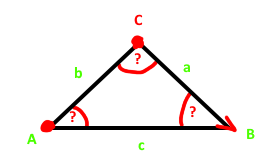# Find Vertex C in triangle 2DWhat would be the most efficient way to find point C, if everything green is given (all edges and Point A and B)?

Well, that’s all just plain math. You can derive the required forumla by using some right angle triangles. Lets label the height of the triangle with h. The height is perpendicular to the side c and hits the side c at point “E”. That point E is x units away from point A. So we get two triangles

``````x² + h² = b² // left triangle
(c-x)² + h² = a² // right triangle
``````

By combining those two we get a solution for x based on a,b and c:

``````x = (b² - a² + c²)/(2*c)
``````

Now that we have x we can simply use the first equation to get h

``````h = sqrt(b² - x²)
``````

Now we have all lengths necessary. All we need now are the normalized direction vectors. We get the vector from A to B by calculating `AB = B - A`. We get point E like this:

``````E = AB.normalized * x;
``````

Once we have E we can “rotate” the direction vector AB 90°. In 2d this is trivial since we just have to swap the x and y coordinates and invert one of them. Which one you invert specifies the rotation direction (clockwise / counter clockwise). With the rotated direction we can simply walk “h” units along that rotated direction from point E upwards or downwards to get to point C

Note that if `x < -b` or `c-x > a` there is no solution as the height can not be calculated as the discriminant inside the square root would be negative. Keep in mind that there are generally two possible solutions for point C. Either “above” the line AB or “below” that line AB. Those two solutions you would get depending on the rotation direction you choose.

I made an interactive Desmos drawing that shows the logic in action. Note that Desmos is a bit picky with variable names. The value “d” is actually “x”. I drawn the two circles that actually intersect which shows the two solutions.